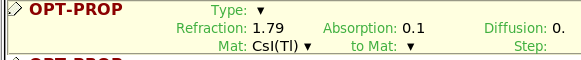# Time consuming in transporting optical photons

Dear experts,

I am trying to count the total optical photons generated by the scintillator according to the following post:

Simulating scintillation response

In the original input file provided by @vojtech.stransky , the refaction index of CsI is set to be 1.0. And the program run normally, except more slowly than the usual calculation.

Then I reassigned the actual refraction index 1.79 to CsI in OPT-PROP card.
And changed the primaries to 1e4.

The program could run normally. However, seems stuck in the transportation in the second primary.
.

Is there something wrong with the reset of refraction index?

Any suggestions are appreciated.

Dear @songhg19 ,

Raising the index of refraction above 1.0 permits total internal reflection. As a consequence, some of the optical photons produced inside the material end up trapped for a very long time. If no mechanism for photon absorption is added to the simulation to effectively limit the survival time of an optical photon you can expect very long simulation runtimes. In the original simulation, the absorption length is infinite. Try raising the absorption coefficients to values higher than 0 and notice how the runtime per primary is decreased. With an absorption coefficient of 0.1 (absorption length of 10 cm), the runtime is about 70 ms/primary. Using a coefficient of 0.01, the runtime is 400 ms/primary!Optical photons can also be absorbed in a reflecting material (declared using “Type=METAL” in the OPT-PROP card). The absorption probability is the value of (1-r).

Dear @blefebvre